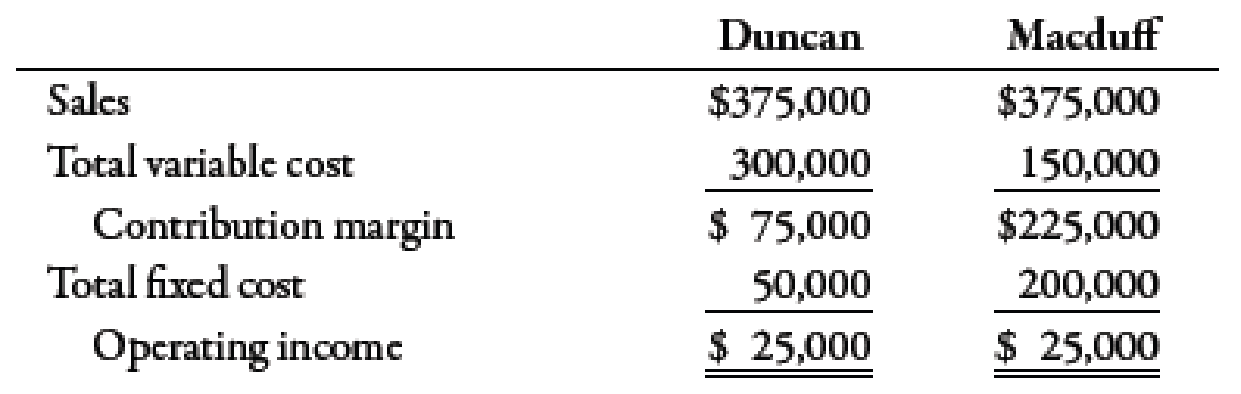Chapter 7, Problem 63P### Managerial Accounting: The Corners...

7th Edition
Maryanne M. Mowen + 2 others
ISBN: 9781337115773

#### Solutions

Chapter
Section### Managerial Accounting: The Corners...

7th Edition
Maryanne M. Mowen + 2 others
ISBN: 9781337115773
Textbook Problem
95 views

# Income statements for two different companies in the same industry are as follows:Required: 1. Compute the degree of operating leverage for each company. 2. CONCEPTUAL CONNECTION Compute the break-even point in dollars for each company. Explain why the break-even point for Macduff is higher. 3. CONCEPTUAL CONNECTION Suppose that both companies experience a 30% increase in revenues. Compute the percentage change in profits for each company. Explain why the percentage increase in Macduff ’s profits is so much larger than that of Duncan.

1.

To determine

Calculate the degree of operating leverage for Company D and Company M.

Explanation

Degree of Operating Leverage (DOL):

The degree of operating leverage can be evaluated by dividing the total contribution margin by the operating income of the company.

Use the following formula to calculate degree of operating leverage for Company D:

Degreeofoperatingleverage=TotalcontributionmarginOperatingincome

Substitute $75,000 for total contribution margin and$25,000 for operating income in the above formula.

Degreeofoperatingleverage=$75,000$25,000=3

Therefore, degree for operating leverage (DOL) is 3

2.

To determine

Calculate break-even point in sales dollars for each company.

3.

To determine

Calculate the percentage increase in profit for each company if there is an increase of 30% in revenues.

### Still sussing out bartleby?

Check out a sample textbook solution.

See a sample solution

#### The Solution to Your Study Problems

Bartleby provides explanations to thousands of textbook problems written by our experts, many with advanced degrees!

Get Started